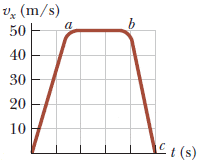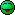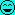# How do i figure out area under the the red-brown line graph?

## Homework Statement

The figure below represents part of the performance data of a car owned by a proud physics student. (The horizontal axis is marked in increments of 2 seconds and the vertical axis is marked in increments of 10 m/s.)(a) Calculate the total distance traveled by computing the area under the red-brown graph line. In kilometers.

## Homework Equations

a = (ΔVx)/Δt
Speed = Total distance/Total Time

## The Attempt at a Solution

I tried to use the trapezoidal method and break it up into 5 increments as I haven't learned how to integrate yet.
Trapezoidal Method
1/2*(f(0) + 2*f(1) + 2*f(2) + 2*f(3) + 2*f(4) + f(5) )
1/2*(f(0) + 2*f(33) + 2*f(50) + 2*f(50) + 2*f(48) + f(0)) = 181 x 10-3 = .181 km
However the answer was a little bigger than what I got which was .375 kilometers.

Last edited by a moderator:

SammyS
Staff Emeritus
Homework Helper
Gold Member

## Homework Statement

The figure below represents part of the performance data of a car owned by a proud physics student. (The horizontal axis is marked in increments of 2 seconds and the vertical axis is marked in increments of 10 m/s.)(a) Calculate the total distance traveled by computing the area under the red-brown graph line. In kilometers.

## Homework Equations

a = (ΔVx)/Δt
Speed = Total distance/Total Time

## The Attempt at a Solution

I tried to use the trapezoidal method and break it up into 5 increments as I haven't learned how to integrate yet.
Trapezoidal Method
1/2*(f(0) + 2*f(1) + 2*f(2) + 2*f(3) + 2*f(4) + f(5) )
1/2*(f(0) + 2*f(33) + 2*f(50) + 2*f(50) + 2*f(48) + f(0)) = 181 x 10-3 = .181 km[/B]
However the answer was a little bigger than what I got which was .375 kilometers.
The region of the graph whose area you're trying to determine is itself approximately a trapezoid.

Find its area.

The region of the graph whose area you're trying to determine is itself approximately a trapezoid.

Find its area.
Would I just need to use the formula (a + AB + BC)/2 * h then? If so what would be a good way to figure out the height?

SteamKing
Staff Emeritus
Homework Helper

## Homework Statement

The figure below represents part of the performance data of a car owned by a proud physics student. (The horizontal axis is marked in increments of 2 seconds and the vertical axis is marked in increments of 10 m/s.)(a) Calculate the total distance traveled by computing the area under the red-brown graph line. In kilometers.

## Homework Equations

a = (ΔVx)/Δt
Speed = Total distance/Total Time

## The Attempt at a Solution

I tried to use the trapezoidal method and break it up into 5 increments as I haven't learned how to integrate yet.
Trapezoidal Method
1/2*(f(0) + 2*f(1) + 2*f(2) + 2*f(3) + 2*f(4) + f(5) )
1/2*(f(0) + 2*f(33) + 2*f(50) + 2*f(50) + 2*f(48) + f(0)) = 181 x 10-3 = .181 km[/B]
However the answer was a little bigger than what I got which was .375 kilometers.
The notation you are using is confusing.

You are given that the horizontal coordinate is time in increments of 2 sec.

If you want to write the ordinates of the curve using functional notation, you would use, for example, f(2) = 33 m/s, not f(33), which suggests you want to find the velocity when t = 33 sec.

As to your application of the trapezoidal rule, you got that mostly correct, except you overlooked that the spacing between your ordinates was 2 sec., not 1 sec.

The trapezoidal formula is A = (1/2) * h * [y1 + 2 * y2 + ... + 2 * yn-1 + yn]

In this instance, h = 2 sec.

Last edited:
epenguin
Homework Helper
Gold Member
If so what would be a good way to figure out the height?

Er, read it off the graph.

You have got there a rectangle and two triangles. The triangles are not the same area as each other though.

The notation you are using is confusing.

You are given that the horizontal coordinate is time in increments of 2 sec.

If you want to write the ordinates of the curve using functional notation, you would use, for example, f(2) = 33 m/s, not f(33), which suggests you want to find the velocity when t = 33 sec.

As to your application of the trapezoidal rule, you got that mostly correct, except you overlooked that the spacing between your ordinates was 2 sec., not 1 sec.

The trapezoidal formula is A = (1/2) * h * [y1 + 2 * y2 + ... + 2 * yn-1 + yn]

In this instance, h = 2 sec.

I think I've got it down now, I have broke it down to (1/2)*2*(0+2(33)+2(50)+2(50)+2(48)+0) which gives me 362 meters, and converted to kilometers it would give me .362 km.

epenguin
Homework Helper
Gold Member
I think I've got it down now, I have broke it down to (1/2)*2*(0+2(33)+2(50)+2(50)+2(48)+0) which gives me 362 meters, and converted to kilometers it would give me .362 km.

You've divided it into five areas, you only needed to divide it into three, see #5, and more seriously you've calculated the triangle areas wrong.

You've divided it into five areas, you only needed to divide it into three, see #5, and more seriously you've calculated the triangle areas wrong.
How exactly did I mess up the areas?

epenguin
Homework Helper
Gold Member
Just look at the first triangle. It's area is not ½ × 2(32) as you have written. The last one is not right either.

Then if you divided into fewer areas as I suggested you would make fewer errors - even if the percentage is the sameSteamKing
Staff Emeritus
Homework Helper
Just look at the first triangle. It's area is not ½ × 2(32) as you have written. The last one is not right either.

Then if you divided into fewer areas as I suggested you would make fewer errors - even if the percentage is the sameI believe the OP is using the trapezoidal rule to calculate the area under this curve:

I think I've got it down now, I have broke it down to (1/2)*2*(0+2(33)+2(50)+2(50)+2(48)+0) which gives me 362 meters, and converted to kilometers it would give me .362 km.

In applying this rule, the ordinates of the curve at evenly spaced intervals are used, rather than calculating triangles and whatnot.

SammyS
Staff Emeritus
Homework Helper
Gold Member
I believe the OP is using the trapezoidal rule to calculate the area under this curve:

In applying this rule, the ordinates of the curve at evenly spaced intervals are used, rather than calculating triangles and whatnot.
I suspect that OP recognized something which looks rather like a trapezoid, then tried to use something called the trapezoid rule.

Yes, I've been trying to use the trapezoid rule

SammyS
Staff Emeritus
Homework Helper
Gold Member
Yes, I've been trying to use the trapezoid rule
Any particular reason for using that rule?

Any particular reason for using that rule?
It was one of the only ways that I knew how to find the area under the line, since I haven't learned how to integrate yet.

SammyS
Staff Emeritus
Homework Helper
Gold Member
It was one of the only ways that I knew how to find the area under the line, since I haven't learned how to integrate yet.
Forget the trapezoid rule, unless you were explicitly instructed to use it.

Except for the rounded corners, you do have a trapezoid, the vertices of which are at
(0, 0), (3, 50), (8. 50), and (10, 0) ,

The x coordinates being in units of seconds, and the y coordinates in units of m/s.

SteamKing
Staff Emeritus
Homework Helper
The trapezoidal rule, while not as elegant as some methods of numerical integration, is a valid technique for calculating the area under this curve:

http://www.math.vt.edu/courses/math2015/Labs/Lab2/TTrap1.html

The trapezoidal rule is first cousin to Simpson's Rule and other rules for integration.

The OP calculated an area of 0.362 km using the trapezoidal rule, and the correct answer, also according to the OP was 0.375 km.

epenguin
Homework Helper
Gold Member
Approximately right answer looks like cancellation of errors to me. Most people who have left school won't remember what a trapezoid even is let alone its rule. I had to look the first up, not bothered with the second.

There you've got a triangle base 3 height 50 area = 3 X 50/2 = 75
A rectangle 5 X 50 Area = 250
A triangle base 2 height 50 area = 2 X 50/2 = 50

+. +. +. = 375

Simples. "Be a country boy".

Approximately right answer looks like cancellation of errors to me. Most people who have left school won't remember what a trapezoid even is let alone its rule. I had to look the first up, not bothered with the second.

There you've got a triangle base 3 height 50 area = 3 X 50/2 = 75
A rectangle 5 X 50 Area = 250
A triangle base 2 height 50 area = 2 X 50/2 = 50

+. +. +. = 375

Simples. "Be a country boy".
Now I see what you did, this method seems a lot simpler than what I was originally thinking.

Forget the trapezoid rule, unless you were explicitly instructed to use it.

Except for the rounded corners, you do have a trapezoid, the vertices of which are at
(0, 0), (3, 50), (8. 50), and (10, 0) ,

The x coordinates being in units of seconds, and the y coordinates in units of m/s.
When I calculate the ΔV/Δt it will give me acceleration which I can then use to find the distance, I think I've got the hang of this now.

SteamKing
Staff Emeritus
Homework Helper
Approximately right answer looks like cancellation of errors to me.
Perhaps, but the curve as shown is not really a true trapezoid either, what with those rounded corners.

Most people who have left school won't remember what a trapezoid even is let alone its rule.

It's OK to admit that the OP caught you flat-footed by using the trapezoidal rule to figure the area under this curve.I had to look the first up, not bothered with the second.
That's too bad, because both rules are rather easy to remember, and each gets used in quite a few situations.

In my line of work, knowing how to use the various rules for numerical integration is second nature, and that includes knowing not only the trapezoidal rule, but also the first and second Simpson's rules and the various Gauss quadrature techniques, as well.•SammyS
epenguin
Homework Helper
Gold Member
Perhaps, but the curve as shown is not really a true trapezoid either, what with those rounded corners.

Reading the OP's first attempts I thought we'd be doing well to help him towards an 8/10 and not be over-ambitious.Best is enemy of good!
Turns out who set the question thought same way.It's OK to admit that the OP caught you flat-footed by using the trapezoidal rule to figure the area under this curve.That's too bad, because both rules are rather easy to remember, and each gets used in quite a few situations.

In my line of work, knowing how to use the various rules for numerical integration is second nature, and that includes knowing not only the trapezoidal rule, but also the first and second Simpson's rules and the various Gauss quadrature techniques, as well.Well useful as you say in your line of work, on the other hand most people won't be there.Useful to more people to have seen them once or twice.

I don't mind not knowing the trapezoid rule, I managed without it. All bings back to me a friend at school who advanced the theory that it was bad to know things, because it made the mind rigid.I sort of still see what he meant. And one could fetch quotations from Einstein and Francis Crick to that effect. But mustn't go further down that line... except to say aren't we dealing here with a lot of students who 'know' things but are blocked in doing anything with them?

The OP seemed to be applying some rules but they were more hindrance than help to him IMHO.

On areas I remember a colleague with a lot of measurements of the sorts of things, radioactive incorporations etc. you measure over a cell cycle in culture who needed the areas. He couldn't remember the formulae. He just cut out silhouettes of the graph paper plots and weighed them. Good a method as any for his and many other purposes.

Last edited:
SteamKing
Staff Emeritus
•